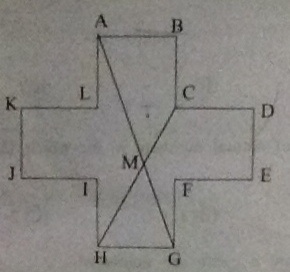# NMTC Inter Level Problem 10

Geometry Level 2Consider the figure $ABCDEFGHIJKL$ as shown. Each side is of length $4$ and the angle between any two consecutive sides is a right angle. $AG$ and $CH$ intersect at $M$. The area of the quadrilateral $ABCM$ is $\text{\_\_\_\_\_\_\_\_\_\_}.$

×